# Boolean Indexing in Pandas

• Difficulty Level : Medium
• Last Updated : 08 Jun, 2022

In boolean indexing, we will select subsets of data based on the actual values of the data in the DataFrame and not on their row/column labels or integer locations. In boolean indexing, we use a boolean vector to filter the data.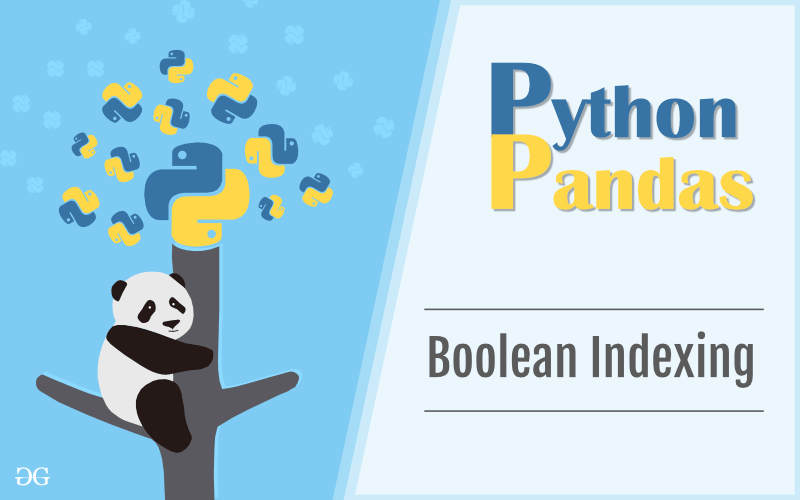Boolean indexing is a type of indexing that uses actual values of the data in the DataFrame. In boolean indexing, we can filter a data in four ways:

• Accessing a DataFrame with a boolean index
• Applying a boolean mask to a dataframe
• Masking data based on column value
• Masking data based on an index value

### Accessing a DataFrame with a boolean index:

In order to access a dataframe with a boolean index, we have to create a dataframe in which the index of dataframe contains a boolean value that is “True” or “False”.

Example

## Python3

 `# importing pandas as pd` `import` `pandas as pd` ` `  `# dictionary of lists` `dict` `=` `{``'name'``:[``"aparna"``, ``"pankaj"``, ``"sudhir"``, ``"Geeku"``],` `        ``'degree'``: [``"MBA"``, ``"BCA"``, ``"M.Tech"``, ``"MBA"``],` `        ``'score'``:[``90``, ``40``, ``80``, ``98``]}` ` `  `df ``=` `pd.DataFrame(``dict``, index ``=` `[``True``, ``False``, ``True``, ``False``])` ` `  `print``(df)`

Output:Now we have created a dataframe with the boolean index after that user can access a dataframe with the help of the boolean index. User can access a dataframe using three functions that is .loc[], .iloc[], .ix[]

### Accessing a Dataframe with a boolean index using .loc[]

In order to access a dataframe with a boolean index using .loc[], we simply pass a boolean value (True or False) in a .loc[] function.

## Python3

 `# importing pandas as pd` `import` `pandas as pd` ` `  `# dictionary of lists` `dict` `=` `{``'name'``:[``"aparna"``, ``"pankaj"``, ``"sudhir"``, ``"Geeku"``],` `        ``'degree'``: [``"MBA"``, ``"BCA"``, ``"M.Tech"``, ``"MBA"``],` `        ``'score'``:[``90``, ``40``, ``80``, ``98``]}`   `# creating a dataframe with boolean index ` `df ``=` `pd.DataFrame(``dict``, index ``=` `[``True``, ``False``, ``True``, ``False``])`   `# accessing a dataframe using .loc[] function ` `print``(df.loc[``True``])`

Output: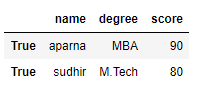### Accessing a Dataframe with a boolean index using .iloc[]

In order to access a dataframe using .iloc[], we have to pass a boolean value (True or False)  but iloc[] function accepts only integer as an argument so it will throw an error so we can only access a dataframe when we pass an integer in iloc[] function

Code #1:

## Python3

 `# importing pandas as pd` `import` `pandas as pd` ` `  `# dictionary of lists` `dict` `=` `{``'name'``:[``"aparna"``, ``"pankaj"``, ``"sudhir"``, ``"Geeku"``],` `        ``'degree'``: [``"MBA"``, ``"BCA"``, ``"M.Tech"``, ``"MBA"``],` `        ``'score'``:[``90``, ``40``, ``80``, ``98``]}`   `# creating a dataframe with boolean index  ` `df ``=` `pd.DataFrame(``dict``, index ``=` `[``True``, ``False``, ``True``, ``False``])`   `# accessing a dataframe using .iloc[] function ` `print``(df.iloc[``True``])`

Output:

`TypeError`

Code #2:

## Python3

 `# importing pandas as pd` `import` `pandas as pd` ` `  `# dictionary of lists` `dict` `=` `{``'name'``:[``"aparna"``, ``"pankaj"``, ``"sudhir"``, ``"Geeku"``],` `        ``'degree'``: [``"MBA"``, ``"BCA"``, ``"M.Tech"``, ``"MBA"``],` `        ``'score'``:[``90``, ``40``, ``80``, ``98``]}`   `# creating a dataframe with boolean index  ` `df ``=` `pd.DataFrame(``dict``, index ``=` `[``True``, ``False``, ``True``, ``False``])` ` `    `# accessing a dataframe using .iloc[] function` `print``(df.iloc[``1``])`

Output: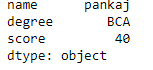### Accessing a Dataframe with a boolean index using .ix[]

In order to access a dataframe using .ix[], we have to pass boolean value (True or False) and integer value to .ix[] function because as we know that .ix[] function is a hybrid of .loc[] and .iloc[] function.

Code #1:

## Python3

 `# importing pandas as pd` `import` `pandas as pd` ` `  `# dictionary of lists` `dict` `=` `{``'name'``:[``"aparna"``, ``"pankaj"``, ``"sudhir"``, ``"Geeku"``],` `        ``'degree'``: [``"MBA"``, ``"BCA"``, ``"M.Tech"``, ``"MBA"``],` `        ``'score'``:[``90``, ``40``, ``80``, ``98``]}`   `# creating a dataframe with boolean index` `df ``=` `pd.DataFrame(``dict``, index ``=` `[``True``, ``False``, ``True``, ``False``])` ` `    `# accessing a dataframe using .ix[] function` `print``(df.ix[``True``])`

Output: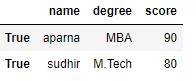Code #2:

## Python

 `# importing pandas as pd` `import` `pandas as pd` ` `  `# dictionary of lists` `dict` `=` `{``'name'``:[``"aparna"``, ``"pankaj"``, ``"sudhir"``, ``"Geeku"``],` `        ``'degree'``: [``"MBA"``, ``"BCA"``, ``"M.Tech"``, ``"MBA"``],` `        ``'score'``:[``90``, ``40``, ``80``, ``98``]}`   `# creating a dataframe with boolean index ` `df ``=` `pd.DataFrame(``dict``, index ``=` `[``True``, ``False``, ``True``, ``False``])` ` `    `# accessing a dataframe using .ix[] function` `print``(df.ix[``1``])`

Output: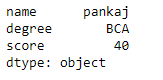### Applying a boolean mask to a dataframe :

In a dataframe, we can apply a boolean mask. In order to do that we can use __getitems__ or [] accessor. We can apply a boolean mask by giving a list of True and False of the same length as contain in a dataframe. When we apply a boolean mask it will print only that dataframe in which we pass a boolean value True. To download “nba1.1” CSV file click here.

Code #1:

## Python3

 `# importing pandas as pd` `import` `pandas as pd` ` `  `# dictionary of lists` `dict` `=` `{``'name'``:[``"aparna"``, ``"pankaj"``, ``"sudhir"``, ``"Geeku"``],` `        ``'degree'``: [``"MBA"``, ``"BCA"``, ``"M.Tech"``, ``"MBA"``],` `        ``'score'``:[``90``, ``40``, ``80``, ``98``]}` ` `  `df ``=` `pd.DataFrame(``dict``, index ``=` `[``0``, ``1``, ``2``, ``3``])` ` `      `print``(df[[``True``, ``False``, ``True``, ``False``]])`

Output: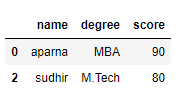Code #2:

## Python3

 `# importing pandas package` `import` `pandas as pd` ` `  `# making data frame from csv file` `data ``=` `pd.read_csv(``"nba1.1.csv"``)` ` `  `df ``=` `pd.DataFrame(data, index ``=` `[``0``, ``1``, ``2``, ``3``, ``4``, ``5``, ``6``,` `                                 ``7``, ``8``, ``9``, ``10``, ``11``, ``12``])`   ` `  `print``(df[[``True``, ``False``, ``True``, ``False``, ``True``,` `    ``False``, ``True``, ``False``, ``True``, ``False``,` `                ``True``, ``False``, ``True``]])`

Output: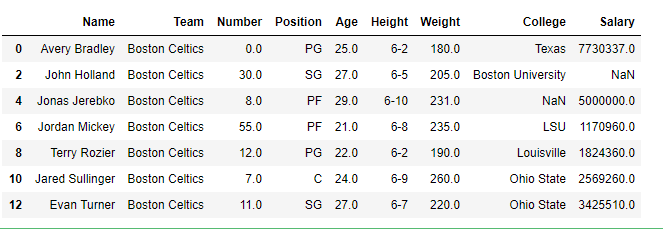### Masking data based on column value:

In a dataframe we can filter a data based on a column value.  In order to filter data, we can apply certain conditions on the dataframe using different operators like ==, >, <, <=, >=. When we apply these operators to the dataframe then it produces a Series of True and False. To download the “nba.csv” CSV, click here.

Code #1:

## Python

 `# importing pandas as pd` `import` `pandas as pd` ` `  `# dictionary of lists` `dict` `=` `{``'name'``:[``"aparna"``, ``"pankaj"``, ``"sudhir"``, ``"Geeku"``],` `        ``'degree'``: [``"BCA"``, ``"BCA"``, ``"M.Tech"``, ``"BCA"``],` `        ``'score'``:[``90``, ``40``, ``80``, ``98``]}`   `# creating a dataframe ` `df ``=` `pd.DataFrame(``dict``)` ` `  `# using a comparison operator for filtering of data` `print``(df[``'degree'``] ``=``=` `'BCA'``)`

Output: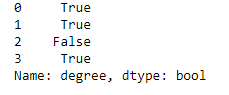Code #2:

## Python

 `# importing pandas package` `import` `pandas as pd` ` `  `# making data frame from csv file` `data ``=` `pd.read_csv(``"nba.csv"``, index_col ``=``"Name"``)` ` `  `# using greater than operator for filtering of data` `print``(data[``'Age'``] > ``25``)`

Output: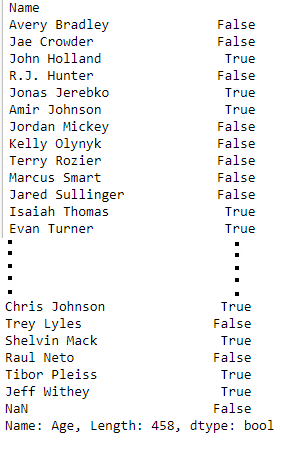Masking data based on index value :

In a dataframe we can filter a data based on a column value. In order to filter data, we can create a mask based on the index values using different operators like ==, >, <, etc… . To download “nba1.1” CSV file click here.

Code #1:

## Python3

 `# importing pandas as pd` `import` `pandas as pd` ` `  `# dictionary of lists` `dict` `=` `{``'name'``:[``"aparna"``, ``"pankaj"``, ``"sudhir"``, ``"Geeku"``],` `        ``'degree'``: [``"BCA"``, ``"BCA"``, ``"M.Tech"``, ``"BCA"``],` `        ``'score'``:[``90``, ``40``, ``80``, ``98``]}` ` `    `df ``=` `pd.DataFrame(``dict``, index ``=` `[``0``, ``1``, ``2``, ``3``])`   `mask ``=` `df.index ``=``=` `0`   `print``(df[mask])`

Output: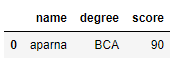Code #2:

## Python3

 `# importing pandas package` `import` `pandas as pd` ` `  `# making data frame from csv file` `data ``=` `pd.read_csv(``"nba1.1.csv"``)`   `# giving a index to a dataframe` `df ``=` `pd.DataFrame(data, index ``=` `[``0``, ``1``, ``2``, ``3``, ``4``, ``5``, ``6``,` `                                 ``7``, ``8``, ``9``, ``10``, ``11``, ``12``])`   `# filtering data on index value` `mask ``=` `df.index > ``7`   `print``(df[mask])`

Output: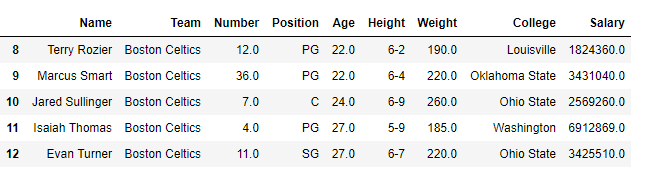My Personal Notes arrow_drop_up
Recommended Articles
Page :